GeeksforGeeks App
Open AppBrowser
Continue

# K means Clustering – Introduction

K-Means Clustering is an Unsupervised Machine Learning algorithm, which groups the unlabeled dataset into different clusters.

## K means Clustering

Unsupervised Machine Learning learning is the process of teaching a computer to use unlabeled, unclassified data and enabling the algorithm to operate on that data without supervision. Without any previous data training, the machine’s job in this case is to organize unsorted data according to parallels, patterns, and variations.

The goal of clustering is to divide the population or set of data points into a number of groups so that the data points within each group are more comparable to one another and different from the data points within the other groups. It is essentially a grouping of things based on how similar and different they are to one another.

We are given a data set of items, with certain features, and values for these features (like a vector). The task is to categorize those items into groups. To achieve this, we will use the K-means algorithm; an unsupervised learning algorithm. ‘K’ in the name of the algorithm represents the number of groups/clusters we want to classify our items into.

(It will help if you think of items as points in an n-dimensional space).  The algorithm will categorize the items into k groups or clusters of similarity. To calculate that similarity, we will use the euclidean distance as a measurement.

The algorithm works as follows:

1. First, we randomly initialize k points, called means or cluster centroids.
2. We categorize each item to its closest mean and we update the mean’s coordinates, which are the averages of the items categorized in that cluster so far.
3. We repeat the process for a given number of iterations and at the end, we have our clusters.

The “points” mentioned above are called means because they are the mean values of the items categorized in them. To initialize these means, we have a lot of options. An intuitive method is to initialize the means at random items in the data set. Another method is to initialize the means at random values between the boundaries of the data set (if for a feature x, the items have values in [0,3], we will initialize the means with values for x at [0,3]).

The above algorithm in pseudocode is as follows:

```Initialize k means with random values

--> For a given number of iterations:

--> Iterate through items:

--> Find the mean closest to the item by calculating
the euclidean distance of the item with each of the means

--> Assign item to mean

--> Update mean by shifting it to the average of the items in that cluster```

### Import the necessary Libraries:

We are importing Numpy for statistical computations, Matplotlib to plot the graph, and make_blobs from sklearn.datasets.

## Python3

 `import` `numpy as np``import` `matplotlib.pyplot as plt``from` `sklearn.datasets ``import` `make_blobs`

## Python3

 `X,y ``=` `make_blobs(n_samples ``=` `500``,n_features ``=` `2``,centers ``=` `3``,random_state ``=` `23``)`` ` `fig ``=` `plt.figure(``0``)``plt.grid(``True``)``plt.scatter(X[:,``0``],X[:,``1``])``plt.show()`

Output: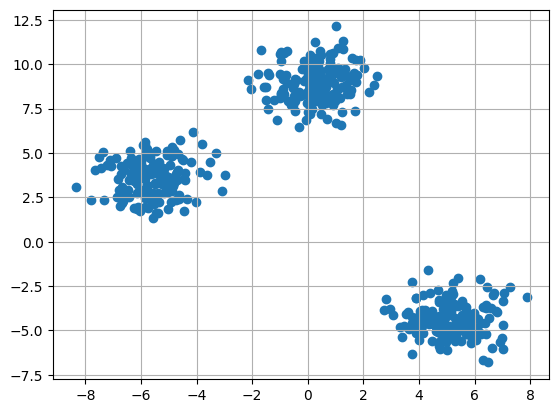Clustering dataset

## Python3

 `k ``=` `3`` ` `clusters ``=` `{}``np.random.seed(``23``)`` ` `for` `idx ``in` `range``(k):``    ``center ``=` `2``*``(``2``*``np.random.random((X.shape[``1``],))``-``1``)``    ``points ``=` `[]``    ``cluster ``=` `{``        ``'center'` `: center,``        ``'points'` `: []``    ``}``     ` `    ``clusters[idx] ``=` `cluster``     ` `clusters`

Output:

```{0: {'center': array([0.06919154, 1.78785042]), 'points': []},
1: {'center': array([ 1.06183904, -0.87041662]), 'points': []},
2: {'center': array([-1.11581855,  0.74488834]), 'points': []}}```

## Python3

 `plt.scatter(X[:,``0``],X[:,``1``])``plt.grid(``True``)``for` `i ``in` `clusters:``    ``center ``=` `clusters[i][``'center'``]``    ``plt.scatter(center[``0``],center[``1``],marker ``=` `'*'``,c ``=` `'red'``)``plt.show()`

Output: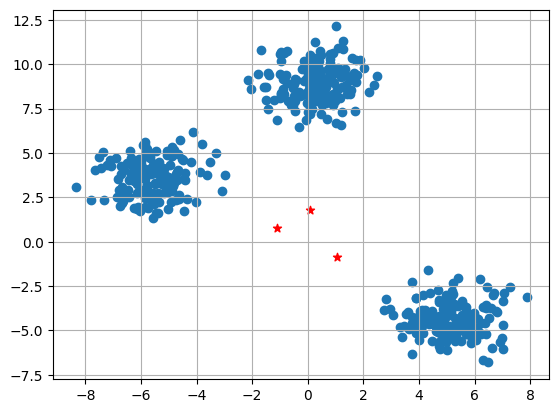Data points with random center

## Python3

 `def` `distance(p1,p2):``    ``return` `np.sqrt(np.``sum``((p1``-``p2)``*``*``2``))`

## Python3

 `#Implementing E step ``def` `assign_clusters(X, clusters):``    ``for` `idx ``in` `range``(X.shape[``0``]):``        ``dist ``=` `[]``         ` `        ``curr_x ``=` `X[idx]``         ` `        ``for` `i ``in` `range``(k):``            ``dis ``=` `distance(curr_x,clusters[i][``'center'``])``            ``dist.append(dis)``        ``curr_cluster ``=` `np.argmin(dist)``        ``clusters[curr_cluster][``'points'``].append(curr_x)``    ``return` `clusters``         ` `#Implementing the M-Step``def` `update_clusters(X, clusters):``    ``for` `i ``in` `range``(k):``        ``points ``=` `np.array(clusters[i][``'points'``])``        ``if` `points.shape[``0``] > ``0``:``            ``new_center ``=` `points.mean(axis ``=``0``)``            ``clusters[i][``'center'``] ``=` `new_center``             ` `            ``clusters[i][``'points'``] ``=` `[]``    ``return` `clusters`

## Python3

 `def` `pred_cluster(X, clusters):``    ``pred ``=` `[]``    ``for` `i ``in` `range``(X.shape[``0``]):``        ``dist ``=` `[]``        ``for` `j ``in` `range``(k):``            ``dist.append(distance(X[i],clusters[j][``'center'``]))``        ``pred.append(np.argmin(dist))``    ``return` `pred`

## Python3

 `clusters ``=` `assign_clusters(X,clusters)``clusters ``=` `update_clusters(X,clusters)``pred ``=` `pred_cluster(X,clusters)`

## Python3

 `plt.scatter(X[:,``0``],X[:,``1``],c ``=` `pred)``for` `i ``in` `clusters:``    ``center ``=` `clusters[i][``'center'``]``    ``plt.scatter(center[``0``],center[``1``],marker ``=` `'^'``,c ``=` `'red'``)``plt.show()`

Output: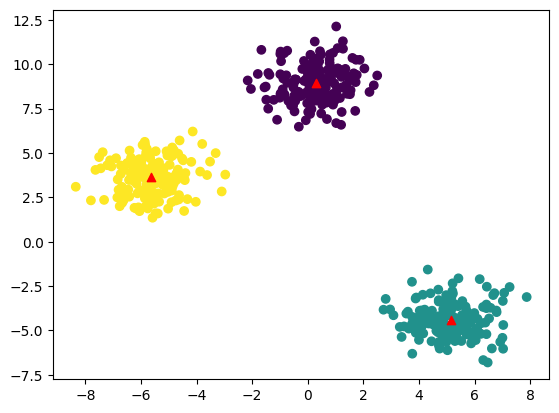K-means Clustering

### Example 2:

Import the necessary libraries

## Python3

 `import` `pandas as pd``import` `numpy as np``import` `seaborn as sns``import` `matplotlib.pyplot as plt``import` `matplotlib.cm as cm``from` `sklearn.datasets ``import` `load_iris``from` `sklearn.cluster ``import` `KMeans`

## Python3

 `X, y ``=` `load_iris(return_X_y``=``True``)`

#### Elbow Method

Finding the ideal number of groups to divide the data into is a basic stage in any unsupervised algorithm. One of the most common techniques for figuring out this ideal value of k is the elbow approach.

## Python3

 `#Find optimum number of cluster``sse ``=` `[] ``#SUM OF SQUARED ERROR``for` `k ``in` `range``(``1``,``11``):``    ``km ``=` `KMeans(n_clusters``=``k, random_state``=``2``)``    ``km.fit(X)``    ``sse.append(km.inertia_)`

## Python3

 `sns.set_style(``"whitegrid"``)``g``=``sns.lineplot(x``=``range``(``1``,``11``), y``=``sse)`` ` `g.``set``(xlabel ``=``"Number of cluster (k)"``, ``      ``ylabel ``=` `"Sum Squared Error"``, ``      ``title ``=``'Elbow Method'``)`` ` `plt.show()`

Output: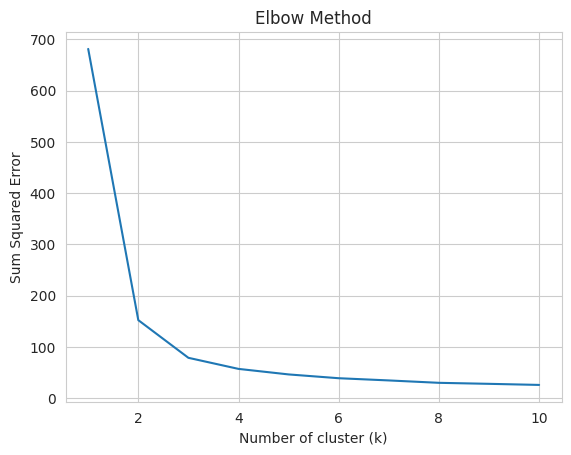Elbow Method

From the above graph, we can observe that at k=2 and k=3 elbow-like situation. So, we are considering K=3

## Python3

 `kmeans ``=` `KMeans(n_clusters ``=` `3``, random_state ``=` `2``)``kmeans.fit(X)`

Output:

```KMeans
KMeans(n_clusters=3, random_state=2)```

## Python3

 `kmeans.cluster_centers_`

Output:

```array([[5.006     , 3.428     , 1.462     , 0.246     ],
[5.9016129 , 2.7483871 , 4.39354839, 1.43387097],
[6.85      , 3.07368421, 5.74210526, 2.07105263]])```

Predict the cluster group:

## Python3

 `pred ``=` `kmeans.fit_predict(X)``pred`

Output:

```array([0, 0, 0, 0, 0, 0, 0, 0, 0, 0, 0, 0, 0, 0, 0, 0, 0, 0, 0, 0, 0, 0,
0, 0, 0, 0, 0, 0, 0, 0, 0, 0, 0, 0, 0, 0, 0, 0, 0, 0, 0, 0, 0, 0,
0, 0, 0, 0, 0, 0, 1, 1, 2, 1, 1, 1, 1, 1, 1, 1, 1, 1, 1, 1, 1, 1,
1, 1, 1, 1, 1, 1, 1, 1, 1, 1, 1, 2, 1, 1, 1, 1, 1, 1, 1, 1, 1, 1,
1, 1, 1, 1, 1, 1, 1, 1, 1, 1, 1, 1, 2, 1, 2, 2, 2, 2, 1, 2, 2, 2,
2, 2, 2, 1, 1, 2, 2, 2, 2, 1, 2, 1, 2, 1, 2, 2, 1, 1, 2, 2, 2, 2,
2, 1, 2, 2, 2, 2, 1, 2, 2, 2, 1, 2, 2, 2, 1, 2, 2, 1], dtype=int32)```

## Python3

 `plt.figure(figsize``=``(``12``,``5``))``plt.subplot(``1``,``2``,``1``)``plt.scatter(X[:,``0``],X[:,``1``],c ``=` `pred, cmap``=``cm.Accent)``plt.grid(``True``)``for` `center ``in` `kmeans.cluster_centers_:``    ``center ``=` `center[:``2``]``    ``plt.scatter(center[``0``],center[``1``],marker ``=` `'^'``,c ``=` `'red'``)``plt.xlabel(``"petal length (cm)"``)``plt.ylabel(``"petal width (cm)"``)``     ` `plt.subplot(``1``,``2``,``2``)   ``plt.scatter(X[:,``2``],X[:,``3``],c ``=` `pred, cmap``=``cm.Accent)``plt.grid(``True``)``for` `center ``in` `kmeans.cluster_centers_:``    ``center ``=` `center[``2``:``4``]``    ``plt.scatter(center[``0``],center[``1``],marker ``=` `'^'``,c ``=` `'red'``)``plt.xlabel(``"sepal length (cm)"``)``plt.ylabel(``"sepal width (cm)"``)``plt.show()`

Output: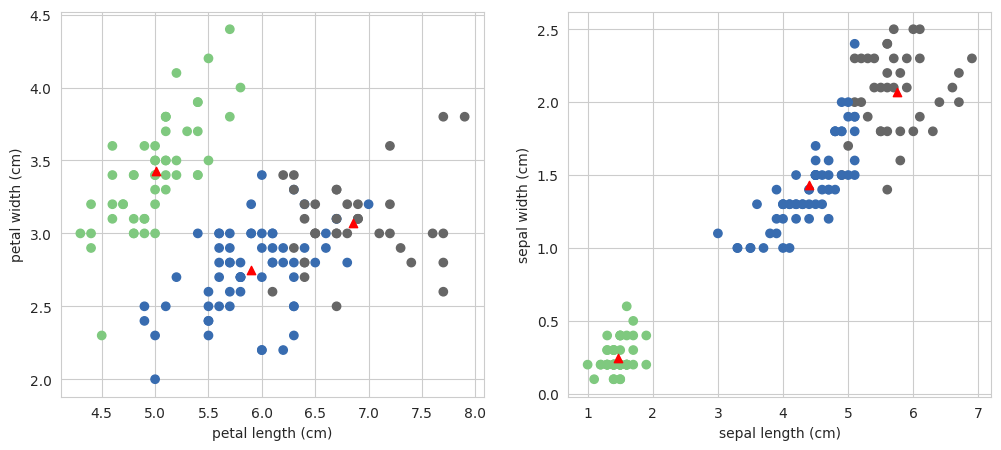K-means clustering

My Personal Notes arrow_drop_up